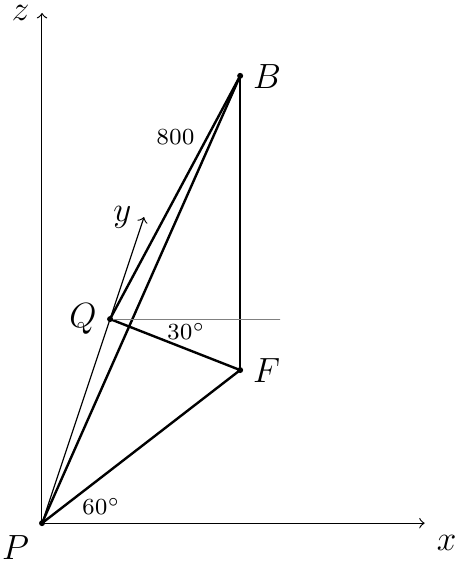# Thread: three-dimensional applications

1. ## three-dimensional applications

A hot-air balloon, B, is observed simultaneously from two points, P and Q, on horizontal ground. From P the bearing of B is 60 degrees at an angle of elevation of 45 degrees. From Q the bearing of B is 330 degrees at an angle of elevation of 60 degrees. The distance BQ is 800 m.

a) Draw a sketch showing the positions of P, Q and B.
b) Calculate the height of the balloon above the ground.
c) Calculate the bearing of Q from P.

Once again I need your help.Time: 2 days.

2.Originally Posted by EftychiaA hot-air balloon, B, is observed simultaneously from two points, P and Q, on horizontal ground. From P the bearing of B is 60 degrees at an angle of elevation of 45 degrees. From Q the bearing of B is 330 degrees at an angle of elevation of 60 degrees. The distance BQ is 800 m.

a) Draw a sketch showing the positions of P, Q and B.
b) Calculate the height of the balloon above the ground.
c) Calculate the bearing of Q from P.

Once again I need your help.Time: 2 days.
1. Draw a sketch.

2. You are dealing actually with 3 right triangles. As the 1st step you can calculate the lengths of the sides of the grey triangle QFB:

$\displaystyle |\overline{QF}|=800 \cdot \cos(60^\circ)$

$\displaystyle |\overline{BF}|=800 \cdot \sin(60^\circ)$

3. Calculate the lengths of the sides of the yellow triangle PFB.

4. Triangle PQF is a right triangle too (why?). Since you know $\displaystyle \overline{PF}$ and $\displaystyle \overline{QF}$ you can calculate the length of $\displaystyle \overline{PQ}$.

5. Calculate the values of the angles $\displaystyle \angle(QPF)$ and $\displaystyle \angle(FQP)$ and then consequently the corresponding bearings.

3. I think the sketch should look like this.4.Originally Posted by emakarovI think the sketch should look like this.
As far as I'm informed bearings are measured clockwise wrt N = 0° = 360°.

Therefore the situation looks like this (see attachment).
I've added the North-direction at P and Q. Add a 3rd parallel line to the North-direction passing through F and you'll see why $\displaystyle \angle(PFQ) = 90^\circ$.

#### Search Tags

applications, threedimensional